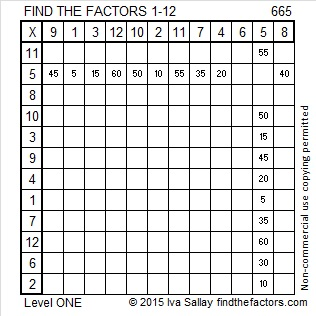# 665 and Level 1

665 is the hypotenuse of the Pythagorean triple 399-532-665. What 3-digit number is the greatest common factor of those three numbers?Print the puzzles or type the solution on this excel file: 12 Factors 2015-11-02

—————————————————————————————————

• 665 is a composite number.
• Prime factorization: 665 = 5 x 7 x 19
• The exponents in the prime factorization are 1, 1, and 1. Adding one to each and multiplying we get (1 + 1)(1 + 1)(1 + 1) = 2 x 2 x 2 = 8. Therefore 665 has exactly 8 factors.
• Factors of 665: 1, 5, 7, 19, 35, 95, 133, 665
• Factor pairs: 665 = 1 x 665, 5 x 133, 7 x 95, or 19 x 35
• 665 has no square factors that allow its square root to be simplified. √665 ≈ 25.78759.—————————————————————————————————This site uses Akismet to reduce spam. Learn how your comment data is processed.